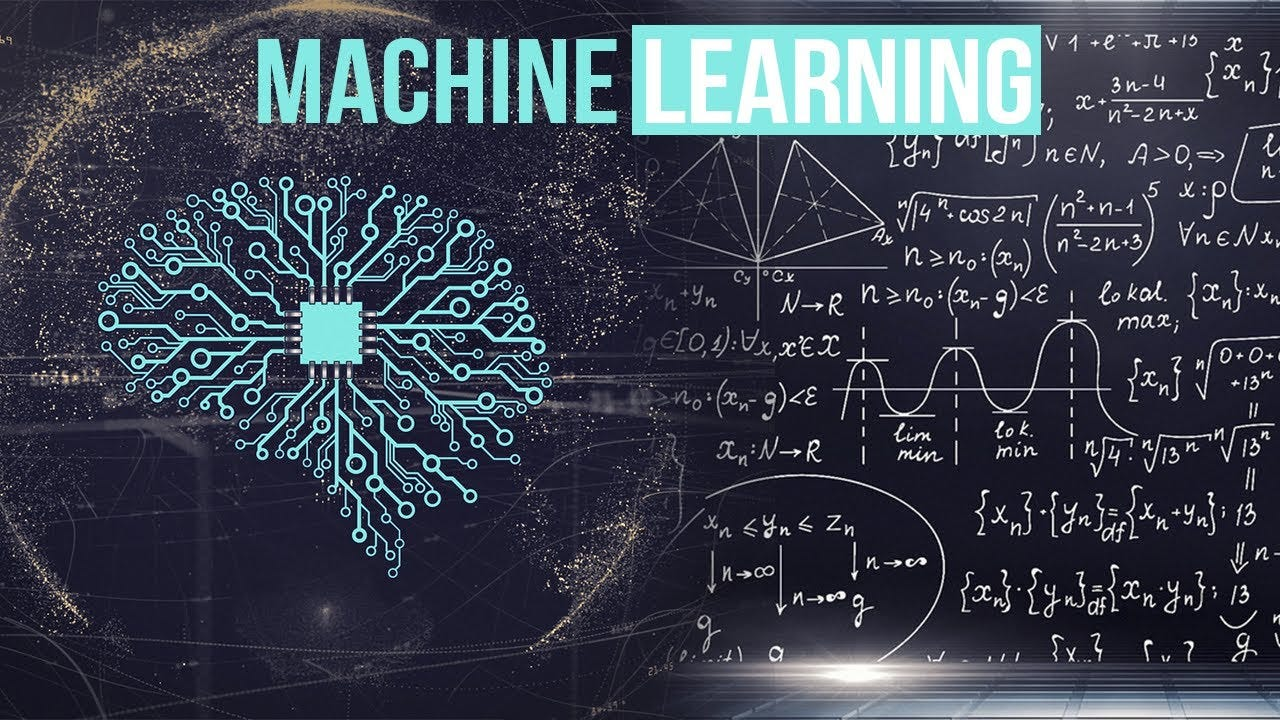# Demystifying the Role of Mathematics in Machine Learning: A Detailed Guide for Aspiring PractitionersA common misconception that prevails among those interested in machine learning (ML) is that a deep understanding of advanced mathematics is a prerequisite to excel in the field. While mathematics is an integral part of machine learning, the extent of its necessity often varies with one’s specific role and the depth of understanding required. This extensive guide will debunk some common myths about the importance of mathematics in machine learning, explore how far one can go without it, and provide useful strategies to conquer mathematical anxiety in this domain.

### The Intersection of Mathematics and Machine Learning

Machine learning, at its core, is a field that originated from statistics and computer science. It involves creating algorithms that can learn from and make predictions or decisions based on data. The fundamental principles of ML are underpinned by various branches of mathematics like statistics, probability, calculus, and linear algebra.

These mathematical theories provide the backbone to algorithms, help in understanding why certain algorithms work better than others, and assist in tweaking algorithms to improve their performance. Therefore, a solid understanding of mathematical concepts can enhance one’s ability to grasp machine learning algorithms’ intricacies.

### Can You Learn Machine Learning Without a Strong Mathematical Background?

The short answer is yes. While understanding the mathematical foundations of ML can be beneficial, it is not an absolute prerequisite, especially for beginners. Here’s why:

1. Abstraction in Modern Libraries: Modern machine learning libraries like TensorFlow, PyTorch, and Scikit-learn provide a high level of abstraction over the raw mathematical operations. This means that you can successfully build and deploy models without getting into the mathematical details.

2. Focus on Practical Application: If you are more interested in applying machine learning rather than developing new algorithms, the emphasis shifts from understanding mathematical derivations to knowing how and when to use different algorithms and techniques.

3. Importance of Data Skills: Proficiency in data manipulation, understanding, and interpretation is often more crucial in real-world ML projects. These skills can be acquired without a deep mathematical background.

### Bridging the Gap: Overcoming Mathematical Anxiety in Machine Learning

If you’re daunted by the mathematical aspects of machine learning, here are some strategies to conquer your fears:

1. Learn On-the-Go: Rather than trying to master all the mathematical concepts upfront, learn them as and when they are needed. This just-in-time learning approach can be more manageable and directly related to the ML concepts you’re learning.

2. Use Visualizations: Mathematical concepts can often be understood more easily when they are visualized. There are numerous online resources available that provide visual explanations of complex mathematical theories.

3. Leverage Online Resources: Today, there are plenty of online courses, video tutorials, and blogs that explain the mathematical aspects of ML in a simplified manner. Resources like Khan Academy, Coursera, and edX offer free or affordable courses on various mathematical topics.

4. Practice, Practice, Practice: As with learning any new skill, practice is key. Implementing ML algorithms from scratch, solving mathematical problems, and taking up ML projects can help solidify your understanding.

5. Join a Community: Engaging with a community of learners can help ease the learning process. You can join forums like Stack Overflow or communities on Reddit, where you can ask questions, share knowledge, and learn from others’ experiences.

### Conclusions

While mathematics is a foundational part of machine learning, not being a math wizard should not deter one from diving into this exciting field. The key is to focus on understanding the practical application of ML algorithms, slowly building up your mathematical knowledge as needed.

With the abundance of learning resources and tools available today, overcoming mathematical anxiety is more attainable than ever. Remember, the path to mastering machine learning isn’t a sprint, but a marathon. As long as you’re patient, persistent, and maintain a willingness to learn, becoming proficient in machine learning is a very achievable goal.

# Personal Career & Learning Guide for Data Analyst, Data Engineer and Data Scientist

## Applied Machine Learning & Data Science Projects and Coding Recipes for Beginners

A list of FREE programming examples together with eTutorials & eBooks @ SETScholars

# Projects and Coding Recipes, eTutorials and eBooks: The best All-in-One resources for Data Analyst, Data Scientist, Machine Learning Engineer and Software Developer

Topics included:Classification, Clustering, Regression, Forecasting, Algorithms, Data Structures, Data Analytics & Data Science, Deep Learning, Machine Learning, Programming Languages and Software Tools & Packages.
(Discount is valid for limited time only)## Find more … …

Python tutorials for Business Analyst – Python Numbers, Type Conversion and Mathematics

Learn Python By Example – Mathematical Operations

Python for Business Analytics – Chapter 9: Simple Mathematical Operators